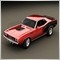Dynamic Array : array out of range11613

Hi, here's a code :

double ArrayHigh[];
double ArrayLow[];
CopyHigh(Symbol(),TStf,0,N,ArrayHigh);
CopyLow(Symbol(),TStf,0,N,ArrayLow);
double LastHeight=0;
double LastPrice=SymbolInfoDouble(Symbol(),SYMBOL_LAST);
for(int i=0; i<N; i++) LastHeight=LastHeight+ArrayHigh[i]-ArrayLow[i];
//--- calculating the average size of the last N bars
LastHeight=LastHeight/N;

With the error I running a test :

2014.10.29 14:08:54     MQL5 Cloud Europe       genetic pass (0, 275) tested with error "critical runtime error 502 in OnTick function (array out of range,  line 721, col 58)" in 16 ms (PR 177)

Any help would be very appreciate !39037

blouf:

Hi, here's a code :

With the error I running a test :

Any help would be very appreciate !

CopyHigh and CopyLow can return less than N value. You have to check the returned value of both functions.11613

angevoyageur:
CopyHigh and CopyLow can return less than N value. You have to check the returned value of both functions.
What's the limitation ? Since I'm trying to optimize ...5631

blouf:
What's the limitation ? Since I'm trying to optimize ...

Try to use something like this:

(if you need at least "N" bars then you should skip these results)

int limit=MathMin(N,ArraySize(ArrayHigh));

for(int i=0; i<limit; i++)11613

lost89:

Try to use something like this:

(if you need at least "N" bars then you should skip these results)

Great ! thanks Lost ! I don't need to do it also with arraylow, supposing it should be the same thing ... right ?5631

blouf:

Great ! thanks Lost ! I don't need to do it also with arraylow, supposing it should be the same thing ... right ?

Yes, it should be the same but you can do the this:

int limit=MathMin(N,MathMin(ArraySize(ArrayHigh),ArraySize(ArrayLow)));